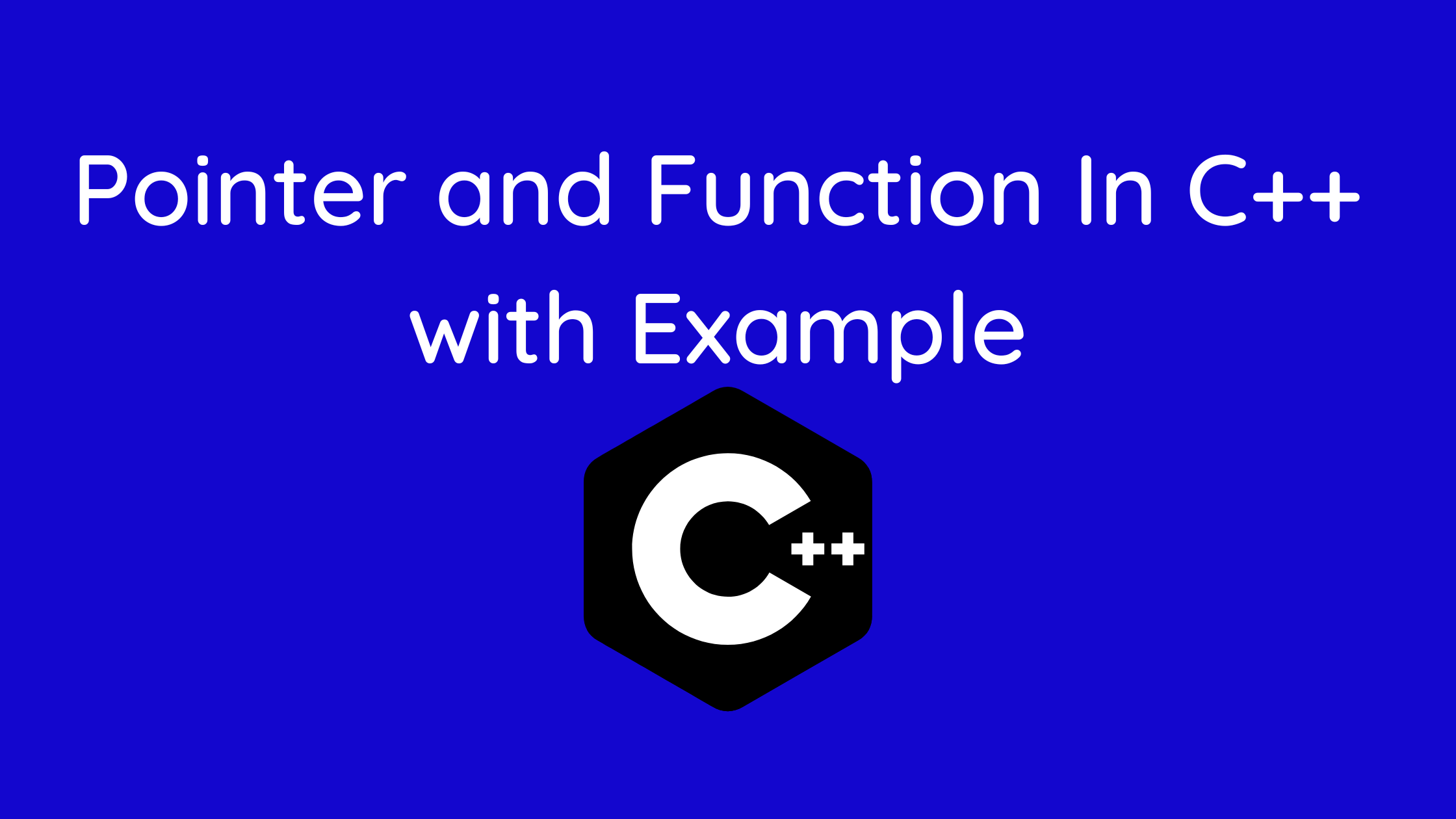## Pointer and Function In C++

With the aid of examples, we will learn about C++ calls by reference and how to give a pointer as an argument to the function.

The function pointer is a pointer used to point to functions, similar to how pointers are used to point to some variables.

Basically, it is employed to save a function’s address. The function pointer can be used to call the function, or the pointer can be passed as an argument to another function.

Passing arguments to a function is a concept covered in the C++ Functions lesson. Because the actual value is sent, the technique utilized is known as passing by value.

The address of an argument is copied into the formal parameter when calling a function using the pointer technique of sending arguments.

The address is utilized within the function to obtain the actual argument used during the call. This implies that adjustments to the parameter will have an impact on the passed argument.

Argument pointers are supplied to the functions in the same manner as other values in order to pass values by the pointer.

To exchange the values of the two integer variables pointed to by its arguments, the following function swap(),

You must specify the function parameters as pointer types.

When a pointer is supplied as a parameter to a function and is then updated, the changes made do not have an effect outside of that function. This is because the function only receives a copy of the pointer.

Passing a pointer by value is equivalent to “pass by pointer,” so to speak. This typically doesn’t pose a problem.

But when you change the pointer inside the code, the issue arises. You are merely altering a duplicate of the pointer; the original pointer is left unaltered. You are not altering the variable.

There is another method of supplying parameters to a function, however, it does not pass the arguments’ real values. The reference to values is passed in its place.

For instance,

```// function that takes value as parameter

void func1(int numVal) {
// code
}

// function that takes reference as parameter
// notice the & before the parameter
void func2(int &numRef) {
// code
}

int main() {
int num = 5;

// pass by value
func1(num);

// pass by reference
func2(num);

return 0;
}```

Take note of the & in void func2 (int &numRef). This indicates that we are utilizing the variable’s address as a parameter.

Therefore, rather than passing the value 5, we are actually passing the address of the num variable when we use the func2() method in main().

Contents

## Example 1: Passing by reference without pointers In Pointer and Function

```#include <iostream>
using namespace std;

// function definition to swap values
void swap(int &n1, int &n2) {
int temp;
temp = n1;
n1 = n2;
n2 = temp;
}

int main()
{

// initialize variables
int a = 1, b = 2;

cout << "Before swapping" << endl;
cout << "a = " << a << endl;
cout << "b = " << b << endl;

// call function to swap numbers
swap(a, b);

cout << "\nAfter swapping" << endl;
cout << "a = " << a << endl;
cout << "b = " << b << endl;

return 0;
}```

## Output

Before swapping
a = 1
b = 2
After swapping
a = 2
b = 1

The variables a and b were supplied to the swap() method in this application. Pay attention to the function definition,

`void swap(int &n1, int &n2)`

& indicates that the function will accept addresses as its parameters in this context.

As a result, the compiler can tell that function parameter are handed references to the variables rather than their real values.

The function parameters n1 and n2 for the swap() function point to the same value as the variables a and b, respectively. As a result, the swapping is based on actual value.

The points can be used to do the same purpose. Visit C++ Pointers to learn more about pointers.

## Example 2: Passing by reference using pointers In Pointer and Function

```#include <iostream>
using namespace std;

// function prototype with pointer as parameters
void swap(int*, int*);

int main()
{

// initialize variables
int a = 1, b = 2;

cout << "Before swapping" << endl;
cout << "a = " << a << endl;
cout << "b = " << b << endl;

// call function by passing variable addresses
swap(&a, &b);

cout << "\nAfter swapping" << endl;
cout << "a = " << a << endl;
cout << "b = " << b << endl;
return 0;
}

// function definition to swap numbers
void swap(int* n1, int* n2) {
int temp;
temp = *n1;
*n1 = *n2;
*n2 = temp;
}```

## Output

Before swapping
a = 1
b = 2
After swapping
a = 2
b = 1

As we can see, this example’s result is identical to that of the preceding one. Observe the line.

```// &a is address of a
// &b is address of b
swap(&a, &b);```

In this case, the variable is not passed during the function call; rather, the variable’s address is.

A dereference operator * must be used to retrieve the value contained in that address since the address is supplied instead of the value.

```temp = *n1;
*n1 = *n2;
*n2 = temp;
```

The values stored at addresses n1 and n2, respectively, are returned by *n1 and *n2.

Any changes made to *n1 and *n2 will alter the actual values of a and b because n1 and n2 hold the addresses of a and b.

As a result, the values of a and b change when we print them in the main() function.